# SAT Math Multiple Choice Question 67: Answer and Explanation

### Test Information

Question: 67

7. If b is two more than one-third of c, which of the following expresses the value of c in terms of b ?

• A. c =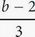• B. c =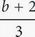• C. c = 3(b - 2)
• D. c = 3(b - 6)

C Whenever there are variables in the question and in the answers, think Plugging In. Let c = 30. Therefore, b = 2 +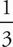(30) = 2 + 10 = 12. Plug 12 in for b in the answers to see which answer equals the target number of 30. Choice (A) becomes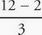=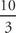=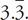Eliminate (A), since it does not equal the target number. Choice (B) becomes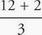=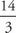=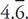Eliminate (B). Choice (C) becomes 3(12 - 2) = 3(10) = 30. Keep (C), but check (D) just in case it also works. Choice (D) becomes 3(12 - 6) = 3(6) = 18. Eliminate (D). The correct answer is (C).